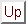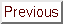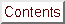Next: Noisy optimization Up: Numerical Results of CONDOR. Previous: Hock and Schittkowski set   Contents

# Parallel results on the Hock and Schittkowski set

We are using the same test conditions as in the previous section (standard objective functions with standard starting points).
Since the objective function is assumed to be time-expensive to evaluate, we can neglect the time spent inside the optimizer and inside the network transmissions. To be able to make this last assumption (negligible network transmissions times), a wait loop of 1 second is embedded inside the code used to evaluate the objective function (only 1 second: to be in the worst case possible).Table 7.2 indicates the number of function evaluations performed on the master CPU (to obtain approximatively the total number of function evaluations cumulated over the master and all the slaves, multiply the given number on the list by the number of CPU's). The CPU time is thus directly proportional to the numbers listed in columns 3 to 5 of the Table 7.2.

Suppose a function evaluation takes 1 hour. The parallel/second process on the main computer has asked 59 minutes ago to a client to perform one such evaluation. We are at step 4(a)i of the main algorithm. We see that there are no new evaluation available from the client computers. Should we go directly to step 4(a)ii and use later this new information, or wait 1 minute? The response is clear: wait a little. This bad situation occurs very often in our test examples since every function evaluation takes exactly the same time (1 second). But what's the best strategy when the objective function is computing, randomly, from 40 to 80 minutes at each evaluation (this is for instance the case for objective functions which are calculated using CFD techniques)? The response is still to investigate. Currently, the implemented strategy is: never wait. Despite, this simple strategy, the current algorithm gives already some non-negligible improvements.Next: Noisy optimization Up: Numerical Results of CONDOR. Previous: Hock and Schittkowski set   Contents
Frank Vanden Berghen 2004-04-19